## Example (2.1): A square raft on irregular subsoil

Introduction

Most of the available solutions used to determine the flexibility coefficient, or the modulus of subgrade reaction, assume that the subsoil consists of a homogeneous layer. In reality, the soil consists of different material features in vertical and horizontal directions. In practice, a number of vertical soil profiles defines the soil under the foundation. Each one has multi-layers with different soil materials. Therefore,three-dimensional coefficient of flexibility, or variable modulus of subgrade reaction, must be taken into consideration. Kany (1972) determined the two-dimensional flexibility coefficient for beam foundation by determining flexibility coefficients for the existing boring logs first. Then, by interpolation can obtain the other coefficients outside the boring logs. This example describes the methods that are available in program ELPLA to determine the three-dimensional coefficient of flexibility or variable modulus of subgrade reaction.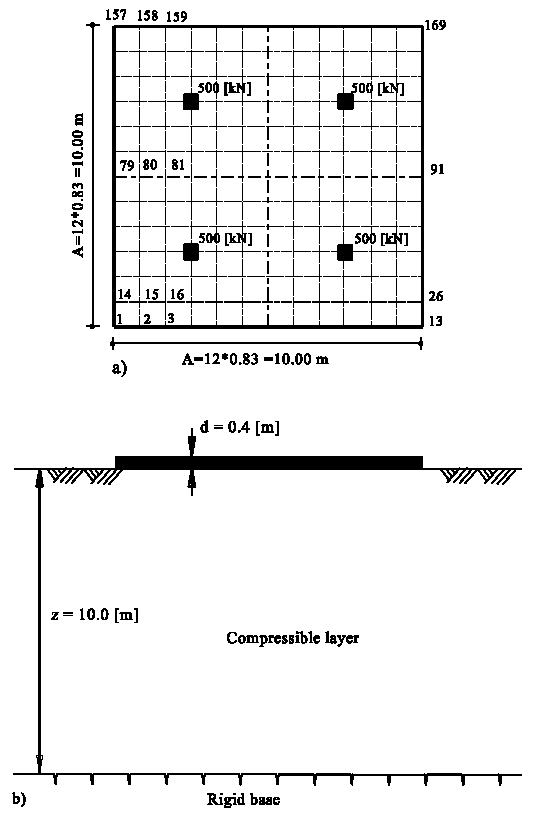Description of the problem

This example is carried out to show the influence of irregular subsoil on the values of settlements, contact pressures and moments. The analysis of the square raft is carried out by the two familiar types of soil models: Winkler's and Continuum models for elastic foundations, besides the analysis of rigid raft on Continuum model, using the following three calculation methods:

Method (3): Variable modulus of subgrade reaction method

Method (7): Modulus of compressibility method

Method (8): Rigid raft on compressible subsoil

A square raft of 10 [m] side is subdivided into 144 square elements as shown in the Figure.

The raft thickness is d = 0.4 [m].

## Example (2.2): An irregular raft on irregular subsoil

Introduction

Most of the available solutions used to determine the flexibility coefficient, or the modulus of subgrade reaction, assume that the subsoil consists of a homogeneous layer. In reality, the soil consists of different material features in vertical and horizontal directions. In practice, a number of vertical soil profiles defines the soil under the foundation. Each one has multi-layers with different soil materials. Therefore, three-dimensional coefficient of flexibility, or variable modulus of subgrade reaction, must be taken into consideration. Kany (1972) determined the two-dimensional flexibility coefficient for beam foundation by determining flexibility coefficients for the existing boring logs first. Then, by interpolation can obtain the other coefficients outside the boring logs. The following paragraph describes the methods that are available in program ELPLA to determine the three-dimensional coefficient of flexibility or variable modulus of subgrade reaction.

Description of the problem

A general example is carried out to show the applicability of the different mathematical models for analysis of irregular rafts on irregular subsoil.

In one case the raft carries many types of external loads: concentrated loads [kN], uniform load [kN/m2], line load [kN/m] and moments [kN.m] in both x-and y-directions as shown in the Figure.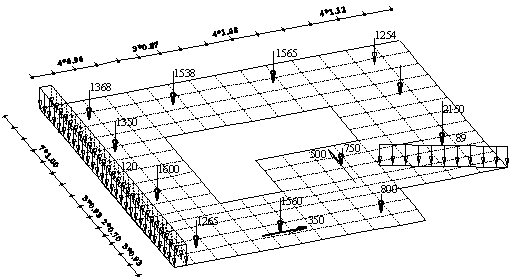## Example (2.3): System of footings on irregular subsoil

Introduction

Most of the available solutions used to determine the flexibility coefficient, or the modulus of subgrade reaction, assume that the subsoil consists of a homogeneous layer. In reality, the soil consists of different material features in vertical and horizontal directions. In practice, a number of vertical soil profiles defines the soil under the foundation. Each one has multi-layers with different soil materials. Therefore,three-dimensional coefficient of flexibility, or variable modulus of subgrade reaction, must be taken into consideration. Kany (1972) determined the two-dimensional flexibility coefficient for beam foundation by determining flexibility coefficients for the existing boring logs first. Then, by interpolation can obtain the other coefficients outside the boring logs. The following paragraph describes the methods that are available in program ELPLA to determine the three-dimensional coefficient of flexibility or variable modulus of subgrade reaction.

Description of the problem

The influence of irregularity of the subsoil material on the behavior of foundations is illustrated through the study of the differential settlements for system of 9 footings. Consider the group of footings shown in the Figure.

Thickness of footings is d = 0.5 [m]. Unit weight of the footing is γf = 25 [kN/m]. Arrangement of footings and footing loads are shown in the Figure (a).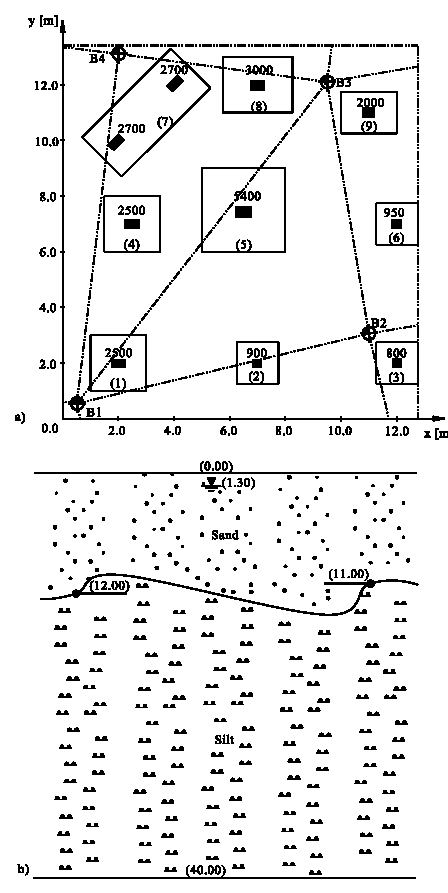## Example (3.1): Settlements outside the foundation

Introduction

In many situations, it becomes important to assess the behavior of a foundation due to its interaction with another neighboring foundation or external load. First, It must be distinguished between two types of problems concerning neighboring foundations:

- The first problem occurs when new building is constructed beside existing one. In this case, the new building will cause an additional settlement under the existing structure due to the increase of stress in soil.

- The second problem occurs when structures are constructed simultaneously. In this case, there will be interaction of foundations due to the overlapping of stresses through the soil medium, however the structures are not statically connected. The interaction of foundations will cause additional settlements under all foundations.

The study of interaction between a foundation and another neighboring foundation or an external load has been considered by several authors. Mikhaiel (1978) presented an application on the use of the elastic half space model in the determination of the effect of neighboring loads on the existing building. Selvadurai (1983) examined the interaction between a rigid circular foundation and an external load. The additional settlement due to neighboring foundation, external loads and buried structures can be considered as follows indicated in the next paragraphs.

Description of the problem

Besides the possibility of studying the influence of neighboring structures on the foundation by the program ELPLA, the described algorithm of ELPLA can be used also for the calculation of settlements outside the foundation. This can be carried out through one of the following two ways:

i) Using a net for the foundation and the unloaded areas outside the foundation. Then, the rigidity of the unloaded areas can be eliminated by assuming very small thickness.

ii) Using two independently nets one for the foundation and the other for the unloaded areas outside the foundation as considered in this example. The Figure shows an irregular raft has the contact area (I) with opening inside it. It is required to determine the settlements at the area (II) around the raft and at the opening of area (III).## Example (3.2): Influence of a new neighboring building on an old one

Introduction

In many situations, it becomes important to assess the behavior of a foundation due to its interaction with another neighboring foundation or external load. First, It must be distinguished between two types of problems concerning neighboring foundations:

- The first problem occurs when new building is constructed beside existing one. In this case, the new building will cause an additional settlement under the existing structure due to the increase of stress in soil.

- The second problem occurs when structures are constructed simultaneously. In this case, there will be interaction of foundations due to the overlapping of stresses through the soil medium, however the structures are not statically connected. The interaction of foundations will cause additional settlements under all foundations.

The study of interaction between a foundation and another neighboring foundation or an external load has been considered by several authors. Mikhaiel (1978) presented an application on the use of the elastic half space model in the determination of the effect of neighboring loads on the existing building. Selvadurai (1983) examined the interaction between a rigid circular foundation and an external load.

The additional settlement due to neighboring foundation, external loads and buried structures can be considered as follows indicated in the next paragraphs.

Description of the problem

For the explanation of the influence of a neighboring building, the influence of a new building on an existing old one is examined in this example.

The Figure shows plan and section of new building (II) beside similar old one (I). The building (I) was constructed since long time, while the building (II) will be constructed close to the first one. The two buildings have the same construction geometry and loads. Also, every building is symmetrical about both x- and y-axes.## Example (3.3): Influence of ground lowering due to a tunnel on a buildingIntroduction

In many situations, it becomes important to assess the behavior of a foundation due to its interaction with another neighboring foundation or external load. First, It must be distinguished between two types of problems concerning neighboring foundations:

- The first problem occurs when new building is constructed beside existing one. In this case, the new building will cause an additional settlement under the existing structure due to the increase of stress in soil.

- The second problem occurs when structures are constructed simultaneously. In this case, there will be interaction of foundations due to the overlapping of stresses through the soil medium, however the structures are not statically connected. The interaction of foundations will cause additional settlements under all foundations.

The study of interaction between a foundation and another neighboring foundation or an external load has been considered by several authors. Mikhaiel (1978) presented an application on the use of the elastic half space model in the determination of the effect of neighboring loads on the existing building. Selvadurai (1983) examined the interaction between a rigid circular foundation and an external load.

The additional settlement due to neighboring foundation, external loads and buried structures can be considered as follows indicated in the next paragraphs.

Description of the problem

The Figure shows a raft of a building consists of two rectangular parts, which are completely connected. The raft is 50 [cm] thick and has a foundation depth of 2.50 [m] under the ground surface. It is planned to construct a tunnel diagonally to the building axis. A primary estimation expects that the tunnel will cause a settlement trough of about 9 [m] width with a maximum lowering of 3 [cm] for the building ground. The settlement trough is plotted in the Figure as contour lines, running symmetrically to the tunnel axis. The influence of the settlement trough due to construction of the tunnel is considered in the analysis of the raft. The raft carries two equal column loads, each of P = 18000 [kN] and line loads of p = 300 [kN/m] from edge walls. The edge walls have 0.30 [m] breadth and 3 [m] height.

## Example (4.1): Interaction of two circular rafts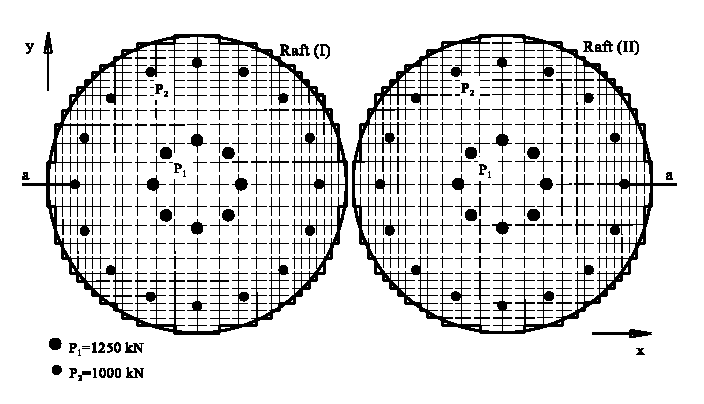Introduction

In many practical cases, it becomes important to study the interaction of elastic or rigid foundations, which are constructed simultaneously. In this case, there will be interaction of foundations due to the overlapping of stresses through the soil medium, however the structures are not statically connected. The interaction of foundations will cause additional settlements under all foundations.

The conventional solution of this problem assumes that the contact pressure of the foundation is known and distributed linearly on the bottom of it. Accordingly, the soil settlements due to the system of foundations can be easily determined. This assumption may be correct for small foundations, but for big foundations, it is preferred to analysis the foundation as a plate resting on either elastic springs (Winkler’s model) or continuum model. In spite of the simplicity of the first model in application, one cannot consider the effect of neighboring foundations or the influence of additional exterior loads. Thus, because Winkler’s model is based on the contact pressure at any point on the bottom of the foundation is proportional to the deflection at that point, independent of the deflections at the other points. Representation of soil as Continuum model (methodes 4, 5, 6, 7 and 8) enables one to consider the effect of external loads.

The study of interaction between a foundation and another neighboring foundation or an external load has been considered by several authors. Stark (1990) presented an example for the interaction between two rafts. Kany (1972) presented an analysis of a system of rigid foundations. In addition, he presented a solution of system of foundations considering the rigidity of the superstructure using a direct method (Kany 1977). Recently, Kany / El Gendy (1997) and (1999) presented an analysis of system of elastic or rigid foundations on irregular subsoil model using an iterative procedure.

This section presents a general solution for the analysis of system of foundations, elastic or rigid, using the iterative procedure of Kany / El Gendy (1997) and (1999).

Description of the problem

To illustrate the application of the iterative procedure of Kany / El Gendy (1997) for the interactive system of foundations, consider the system of two equal large circular rafts shown in the Figure. The rafts rest on a soil layer of thickness 15 [m]. Each raft has a diameter of 22 [m] and 0.65 [m] thickness. Loading on each raft consists of 24 column loads in which 16 columns loads have P1 = 1250 [kN] and 8 column loads have P2 = 1000 [kN]. The Young’s modulus of the raft material is Eb = 2.6×10 [kN/m ] and Poisson’s ratio is νb = 0.15 [-], while the soil values are Es = 9500 [kN/m] and νs = 0 [-].

## Example (4.2): Settlement behavior of four containersIntroduction

In many practical cases, it becomes important to study the interaction of elastic or rigid foundations, which are constructed simultaneously. In this case, there will be interaction of foundations due to the overlapping of stresses through the soil medium, however the structures are not statically connected. The interaction of foundations will cause additional settlements under all foundations.

The conventional solution of this problem assumes that the contact pressure of the foundation is known and distributed linearly on the bottom of it. Accordingly, the soil settlements due to the system of foundations can be easily determined. This assumption may be correct for small foundations, but for big foundations, it is preferred to analysis the foundation as a plate resting on either elastic springs (Winkler’s model) or continuum model. In spite of the simplicity of the first model in application, one cannot consider the effect of neighboring foundations or the influence of additional exterior loads. Thus, because Winkler’s model is based on the contact pressure at any point on the bottom of the foundation is proportional to the deflection at that point, independent of the deflections at the other points. Representation of soil as Continuum model (methodes 4, 5, 6, 7 and 8) enables one to consider the effect of external loads.

The study of interaction between a foundation and another neighboring foundation or an external load has been considered by several authors. Stark (1990) presented an example for the interaction between two rafts. Kany (1972) presented an analysis of a system of rigid foundations. In addition, he presented a solution of system of foundations considering the rigidity of the superstructure using a direct method (Kany 1977). Recently, Kany / El Gendy (1997) and (1999) presented an analysis of system of elastic or rigid foundations on irregular subsoil model using an iterative procedure.

This section presents a general solution for the analysis of system of foundations, elastic or rigid, using the iterative procedure of Kany / El Gendy (1997) and (1999).

Description of the problem

To verify the iterative procedure of Kany / El Gendy (1997) and evaluate its accuracy for interactive large system of rigid rafts, consider the example 2 in the User’s Guide of program STAPLA (Kany (1976)). The computed settlements obtained from the iterative procedure are compared with those of program STAPLA (Kany (1976)).

For a sewerage station, two isolated containers A and B were constructed simultaneously. Then, lately to extend the station another two isolated containers C and D would be constructed at the same area. Those two external C and D containers would provide an additional settlement on containers A and B.

It is required to assess the tilting of each container and the settlement considering the interaction between the containers through the subsoil at the end of construction. The tilting and settlement of the containers are main factors for designing of the pipe connections. The Figure shows the location of containers to each other and the soil layers. The moduli of compressibility of the soil layers for loading and reloading parts are taken as the same Es. Ws.

## Example (4.3): interaction of two rafts considering two additional footings

Introduction

In many practical cases, it becomes important to study the interaction of elastic or rigid foundations, which are constructed simultaneously. In this case, there will be interaction of foundations due to the overlapping of stresses through the soil medium, however the structures are not statically connected. The interaction of foundations will cause additional settlements under all foundations.

The conventional solution of this problem assumes that the contact pressure of the foundation is known and distributed linearly on the bottom of it. Accordingly, the soil settlements due to the system of foundations can be easily determined. This assumption may be correct for small foundations, but for big foundations, it is preferred to analysis the foundation as a plate resting on either elastic springs (Winkler’s model) or continuum model. In spite of the simplicity of the first model in application, one cannot consider the effect of neighboring foundations or the influence of additional exterior loads. Thus, because Winkler’s model is based on the contact pressure at any point on the bottom of the foundation is proportional to the deflection at that point, independent of the deflections at the other points. Representation of soil as Continuum model (methodes 4, 5, 6, 7 and 8) enables one to consider the effect of external loads.

The study of interaction between a foundation and another neighboring foundation or an external load has been considered by several authors. Stark (1990) presented an example for the interaction between two rafts. Kany (1972) presented an analysis of a system of rigid foundations. In addition, he presented a solution of system of foundations considering the rigidity of the superstructure using a direct method (Kany 1977). Recently, Kany / El Gendy (1997) and (1999) presented an analysis of system of elastic or rigid foundations on irregular subsoil model using an iterative procedure.

This section presents a general solution for the analysis of system of foundations, elastic or rigid, using the iterative procedure of Kany / El Gendy (1997) and (1999).

Description of the problem

Besides, the possibility of analysis of large foundation system with many elements by the procedure of Kany / El Gendy (1997), the mesh of the rigid foundation can be generated in analog mode to the finite element mesh of the elastic foundation in one program. Comparing results from analysis of system of rigid rafts with those of elastic or flexible rafts with the same input data is possible. Subsequently the results of the three analyses are compared in an example.

In this example, the settlements of structures due to interactive analysis of system of rigid, elastic and flexible rafts are studied. This example is chosen from the reference Graßhoff / Kany (1997). System of two large rafts and additional two external footings are constructed near each other. The dimensions are shown in the Figures.

## Example (4.4): interaction of two square rafts constructed side by side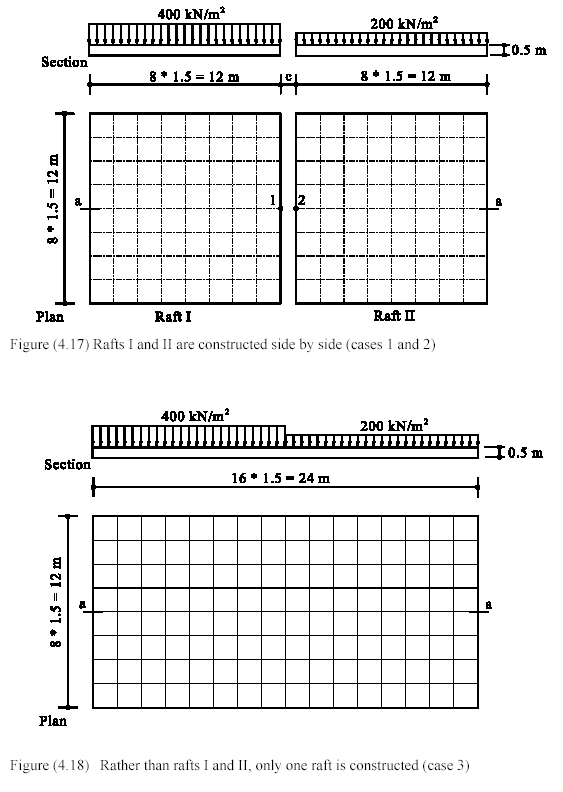Introduction

In many practical cases, it becomes important to study the interaction of elastic or rigid foundations, which are constructed simultaneously. In this case, there will be interaction of foundations due to the overlapping of stresses through the soil medium, however the structures are not statically connected. The interaction of foundations will cause additional settlements under all foundations.

The conventional solution of this problem assumes that the contact pressure of the foundation is known and distributed linearly on the bottom of it. Accordingly, the soil settlements due to the system of foundations can be easily determined. This assumption may be correct for small foundations, but for big foundations, it is preferred to analysis the foundation as a plate resting on either elastic springs (Winkler’s model) or continuum model. In spite of the simplicity of the first model in application, one cannot consider the effect of neighboring foundations or the influence of additional exterior loads. Thus, because Winkler’s model is based on the contact pressure at any point on the bottom of the foundation is proportional to the deflection at that point, independent of the deflections at the other points. Representation of soil as Continuum model (methodes 4, 5, 6, 7 and 8) enables one to consider the effect of external loads.

The study of interaction between a foundation and another neighboring foundation or an external load has been considered by several authors. Stark (1990) presented an example for the interaction between two rafts. Kany (1972) presented an analysis of a system of rigid foundations. In addition, he presented a solution of system of foundations considering the rigidity of the superstructure using a direct method (Kany 1977). Recently, Kany / El Gendy (1997) and (1999) presented an analysis of system of elastic or rigid foundations on irregular subsoil model using an iterative procedure.

This section presents a general solution for the analysis of system of foundations, elastic or rigid, using the iterative procedure of Kany / El Gendy (1997) and (1999).

Description of the problem

Settlement joints are usually used in the foundation when the intensity of loads on it differs considerably from area to another. In such case, the foundation may be divided corresponding to its load intensity to avoid cracks. Settlement joint is constructed by making a complete separated joint in the foundation or a hinged joint. If the foundation has a separated joint, each part will settle independently but it will be interaction between parts of the foundation through the subsoil. In the other case of hinged joint, there will be transmission of shearing forces between connection parts.

This example is carried out to examine the interaction of two rafts considering settlement joint. Consider two equal square rafts I and II will be constructed side by side. Each raft has a side of 12 [m] and 0.5 [m] thickness. Raft I is subject to a uniform load of 400 [kN/m2], while raft II carries a uniform load of 200 [kN/m2].

## Example (4.5): Analysis of a swimming pool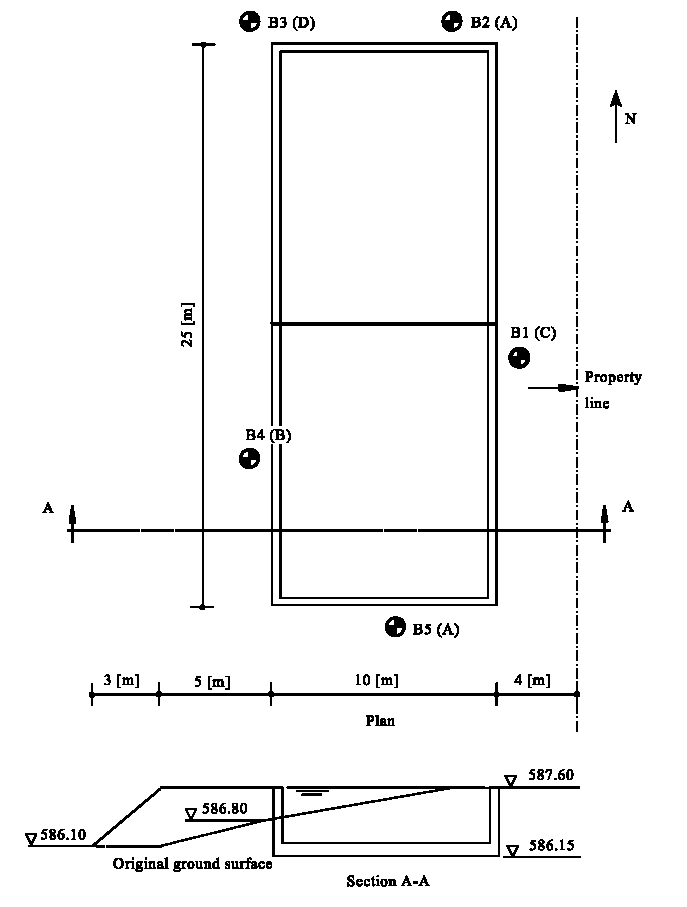Introduction

In many practical cases, it becomes important to study the interaction of elastic or rigid foundations, which are constructed simultaneously. In this case, there will be interaction of foundations due to the overlapping of stresses through the soil medium, however the structures are not statically connected. The interaction of foundations will cause additional settlements under all foundations. The conventional solution of this problem assumes that the contact pressure of the foundation is known and distributed linearly on the bottom of it. Accordingly, the soil settlements due to the system of foundations can be easily determined. This assumption may be correct for small foundations, but for big foundations, it is preferred to analysis the foundation as a plate resting on either elastic springs (Winkler’s model) or continuum model. In spite of the simplicity of the first model in application, one cannot consider the effect of neighboring foundations or the influence of additional exterior loads. Thus, because Winkler’s model is based on the contact pressure at any point on the bottom of the foundation is proportional to the deflection at that point, independent of the deflections at the other points. Representation of soil as Continuum model (methodes 4, 5, 6, 7 and 8) enables one to consider the effect of external loads.

The study of interaction between a foundation and another neighboring foundation or an external load has been considered by several authors. Stark (1990) presented an example for the interaction between two rafts. Kany (1972) presented an analysis of a system of rigid foundations. In addition, he presented a solution of system of foundations considering the rigidity of the superstructure using a direct method (Kany 1977). Recently, Kany/ El Gendy (1997) and (1999) presented an analysis of system of elastic or rigid foundations on irregular subsoil model using an iterative procedure.

This section presents a general solution for the analysis of system of foundations, elastic or rigid, using the iterative procedure of Kany/ El Gendy (1997) and (1999).

Description of the problem

A swimming pool is supposed to be constructed at a river. The existing ground around the pool has to be increased up to a meter. The pool has dimensions of 25 [m] × 10 [m] and maximum water depth of 1.20 [m] as shown in Figure (4.32). The foundation level is 1.45 [m] under the ground surface. Slab and walls are reinforced concrete of concrete grade B 25 with thickness of 25 [cm] for slab and 20 [cm] for walls. It is divided into two independent parts through a joint at the pool middle.

The filling material around the pool is noncohesive soil (Figures (4.33) and (4.34)). The filling is supposed to be carried out after finishing the pool.

In this example, it is required to study the following:

i) Influence of the joint on the settlements, contact pressures and internal forces of the pool slab and the pool walls in case of the pool is completely filled by water.

ii) Influence of the ground rising by additional filling soil material at the southern part of the pool on the settlement.

## Example (5.1): Rigidity of simple square raftIntroduction

The foundation is considered as rigid, elastic or flexible, depends on the ratio between the rigidity of the foundation and the soil. The oldest work for the analysis of foundation rigidity is that of Borowicka (1939). He analyzed the problem of distribution of contact stress under uniformly loaded strip and circular rigid foundations resting on semi-infinite elastic mass.

After Borowicka’s analysis, many authors introduced formulae to find the foundation rigidity for plates resting on different subsoil models. For examples, Gorbunov/ Posadov (1959) introduced formula for an elastic solid medium. Cheung/ Zienkiewicz (1965) introduced formulae for Winkler springs and isotropic elastic half space model. Vlazov/ Leontiv (1966) introduced formula for a two-parameter elastic medium. A good review for those formulae may be found in Selvadurai (1979).

Lately, based on great number of comparative computations for the modulus of compressibility method, Graßhoff (1987) proposed various degrees of system rigidity between foundation and the soil until case of practical rigidity using Equation (5.2). The equation still used in many national standard specifications such as German standard (DIN 4018) and Egyptian Code of Practice (ECP 196-1995).

Description of problem

For comparison with complex foundation rigidity problems, no solution is yet available. Therefore, for judgment on the analysis of El Gendy (1998) to find the system rigidity of foundation, consider the simple example of raft foundation shown in Figure (5.2). The raft has dimensions of 12 [m] × 12 [m] and carries four symmetrical and equal loads, each of P = 9000 [kN]. The raft rests on a homogenous soil layer of thickness 20 [m]. The Young’s modulus of the raft and soil materials are Eb = 2×10 [kN/m] and Es = 10000 [kN/m], respectively. Poisson's ratio of the raft material is νb = 0.15.

## Example (5.2): Rigidity of irregular raft on irregular subsoil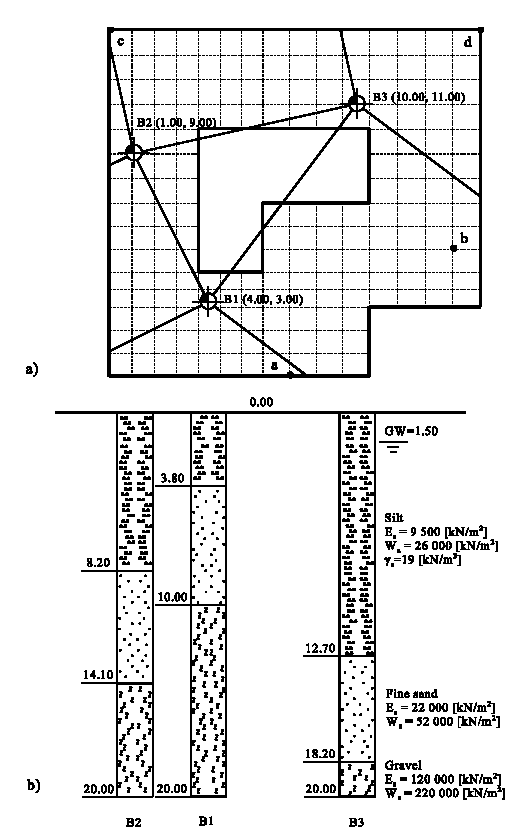Introduction

The foundation is considered as rigid, elastic or flexible, depends on the ratio between the rigidity of the foundation and the soil. The oldest work for the analysis of foundation rigidity is that of Borowicka (1939). He analyzed the problem of distribution of contact stress under uniformly loaded strip and circular rigid foundations resting on semi-infinite elastic mass. After Borowicka’s analysis, many authors introduced formulae to find the foundation rigidity for plates resting on different subsoil models. For examples, Gorbunov/ Posadov (1959) introduced formula for an elastic solid medium. Cheung/ Zienkiewicz (1965) introduced formulae for Winkler springs and isotropic elastic half space model. Vlazov/ Leontiv (1966) introduced formula for a two-parameter elastic medium. A good review for those formulae may be found in Selvadurai (1979).

Lately, based on great number of comparative computations for the modulus of compressibility method, Graßhoff (1987) proposed various degrees of system rigidity between foundation and the soil until case of practical rigidity using Equation (5.2). The equation still used in many national standard specifications such as German standard (DIN 4018) and Egyptian Code of Practice (ECP 196-1995).

Description of problem

A general numerical example is carried out to show the applicability of system rigidity analysis, which proposed by El Gendy (1998), to find the rigid thickness of rafts of any shape considering re-entrant corner and opening within the rafts.

In one case the raft carries many types of external loads; concentrated loads, distributed load, line load and moments in x-and y-direction as shown in The Figure. The raft parameters are Young's modulus Eb = 2×10 [kN/m] and Poisson's ratio νb = 0.25. The level of foundation is df = 2.7 [m].

## Example (5.3): Effect of girders on the raft rigidityIntroduction

The foundation is considered as rigid, elastic or flexible, depends on the ratio between the rigidity of the foundation and the soil. The oldest work for the analysis of foundation rigidity is that of Borowicka (1939). He analyzed the problem of distribution of contact stress under uniformly loaded strip and circular rigid foundations resting on semi-infinite elastic mass.

After Borowicka’s analysis, many authors introduced formulae to find the foundation rigidity for plates resting on different subsoil models. For examples, Gorbunov/ Posadov (1959) introduced formula for an elastic solid medium. Cheung/ Zienkiewicz (1965) introduced formulae for Winkler springs and isotropic elastic half space model. Vlazov/ Leontiv (1966) introduced formula for a two-parameter elastic medium. A good review for those formulae may be found in Selvadurai (1979).

Lately, based on great number of comparative computations for the modulus of compressibility method, Graßhoff (1987) proposed various degrees of system rigidity between foundation and the soil until case of practical rigidity using Equation (5.2). The equation still used in many national standard specifications such as German standard (DIN 4018) and Egyptian Code of Practice (ECP 196-1995).

Description of the problem

Ribbed raft may be used for many structures have heavy loads or large spans, if a flat level for the first floor is not required. Consequently, concrete is reduced. Such structures are silos and elevated tanks. In spite of this type of foundation has many disadvantages if used in normally buildings, still used by many designers. Such disadvantages are the raft needs deep foundation level under the ground surface, fill material on the foundation to make a flat level and an additional slab on the fill material to construct the first floor. The use of the ribbed raft relates to the simplicity of analysis by hand calculations.

First, both of the two rafts with and without ribs are clearly saves and correct, but there is still a question, whose one of the two types is more rigid? To answer this question the following example is presented. Consider the foundation of an elevated tank may be designed for both types of foundations. The foundation has the dimensions of 20 [m] × 20 [m], transmits equal loads for all 25 columns, each of 1000 [kN]. The loads give average contact pressure on soil qav = 62.5 [kN/m]. Columns are equally spaced, 4 [m] apart, in each direction as shown in the Figure.

## Example (5.4): Comparison between raft and grid foundations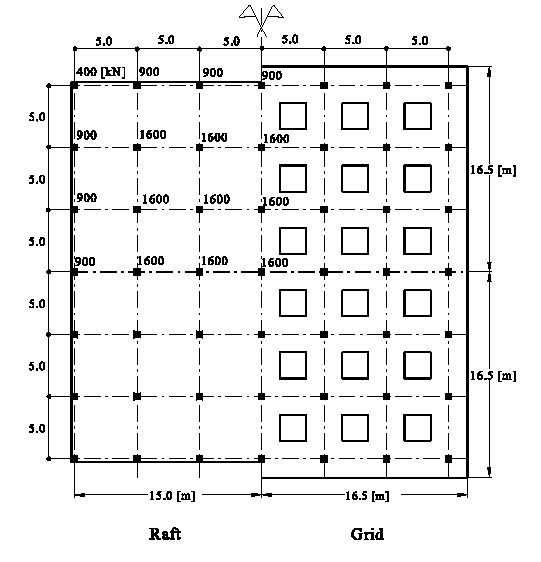Introduction

The foundation is considered as rigid, elastic or flexible, depends on the ratio between the rigidity of the foundation and the soil. The oldest work for the analysis of foundation rigidity is that of Borowicka (1939). He analyzed the problem of distribution of contact stress under uniformly loaded strip and circular rigid foundations resting on semi-infinite elastic mass.

After Borowicka’s analysis, many authors introduced formulae to find the foundation rigidity for plates resting on different subsoil models. For examples, Gorbunov/ Posadov (1959) introduced formula for an elastic solid medium. Cheung/ Zienkiewicz (1965) introduced formulae for Winkler springs and isotropic elastic half space model. Vlazov/ Leontiv (1966) introduced formula for a two-parameter elastic medium. A good review for those formulae may be found in Selvadurai (1979).

Lately, based on great number of comparative computations for the modulus of compressibility method, Graßhoff (1987) proposed various degrees of system rigidity between foundation and the soil until case of practical rigidity using Equation (5.2). The equation still used in many national standard specifications such as German standard (DIN 4018) and Egyptian Code of Practice (ECP 196-1995).

Description of the problem

El Arabi / El Gendy (2001) examined the structural analysis and design of the three common foundation systems: raft, grid and isolated footings. They carried out the examination to evaluate the different types of structural systems in order to decide the most suitable ones for a specific situation. Here, an example is chosen from the above study with some modifications.

Consider the foundation system shown in the Figure, which may be designed as raft or grid. The raft dimensions are 30.5 [m] × 30.5 [m] while the overall grid dimensions are 33.0 [m] × 33.0 [m], with a constant strip width in both directions. The foundation carries 49 column loads, which are equally spaced, 5.0 [m] apart, in each direction. Column loads and the arrangement of columns are shown also in the Figure.

## Example (6.1): Analysis and design of a raft for a high rise building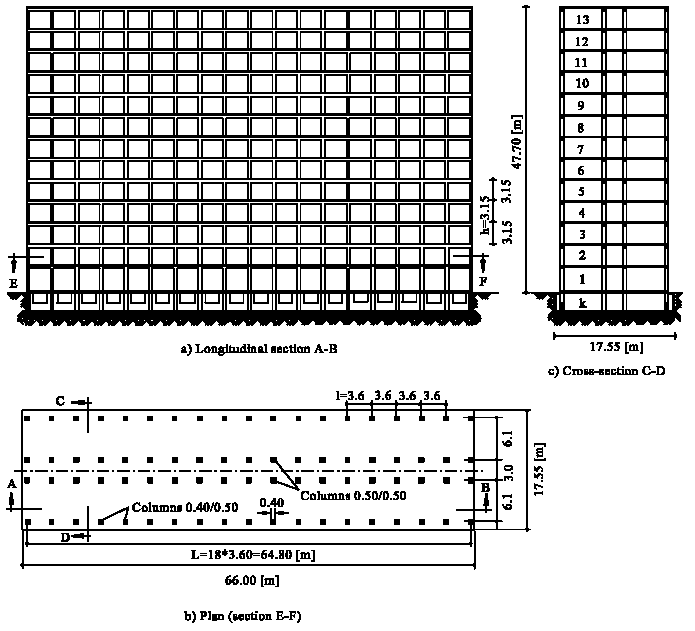Introduction

The presence of the structure on compressible subsoil causes settlements for the foundation and also for the structure itself. Values of settlements and settlement differences depend not only on the thickness of the compressible soil layer under the foundation, the value and distribution of structure loads, the foundation depth and contact pressure under the foundations but also on the flexural rigidity of the structure.

One of the properties that has a considerable influence on the development of settlement is the rigidity of the superstructure. The more rigid structure has more uniform settlement and conversely, structure that is more flexible has greatest difference in settlement. The entire structure can be defined as the three media: superstructure, foundation and soil. The analysis of the entire structure as one unit is very important to find the deformations and internal forces.

However, most of the practical analyses of structures neglect the interaction among the three media to avoid the three-dimensional analysis and modeling. The structure is designed on the assumption of non-displaceable supports while the foundation is designed on the assumption that there is no connection between columns. Such accurate analysis of the entire structure is extremely complex.

The early studies for consideration the effect of the superstructure were by Meyerhof (1953) who suggested an approximate method to evaluate the equivalent stiffness that includes the combined effect of the superstructure and the strip beam foundation. Kany (1959) gave the flexural rigidity of a multi-storey frame structure by an empirical formulae. Also, Kany (1977) analyzed the structure with foundation using a direct method. Demeneghi (1981) used the stiffness method in the structural analysis. Panayotounakos/ Spyropoulos/ Prassianakis (1987) presented an exact matrix solution for the static analysis of a multi-storey and multi-column rectangular plexus frame on an elastic foundation in the most general case of response and loading.

At the analysis of foundations with considering the superstructure stiffness, it is required to distinguish between the analysis for plane structures (two-dimensional analysis) and that for space structures (three-dimensional analysis). Further, it is required to distinguish between approximation methods with closed form equations (Kany (1974), Meyerhof (1953), Sommer (1972)) and refined methods such as conventional plane or space frame analysis (Kany (1976)), Finite Elements (Meyer (1977), Ellner/ Kany (1976), Zilch (1993), Kany/ El Gendy (2000)) or Finite Differences (Bowles (1974), Deninger (1964)).

In addition, many analytical methods are reported for analysis of the entire structure as one unit by using the finite element.

For examples:

Haddadin (1971) presented an explicit program for the analysis of the raft on Winkler's foundation including the effects of superstructure rigidity.

Lee/ Browen (1972) analyzed a plane frame on a two-dimensional foundation.

Hain/ Lee (1974) employed the finite element method to analyze the flexural behavior of a flexible raft foundation taking into account stiffness effect of a framed superstructure. They proposed the use of substructure techniques with finite element formulation to model space frame-raft-soil systems. The supporting soil was represented by either of two types of soil models (Winkler and half-space models).

Poulos (1975) formulated the interaction of superstructure and foundation by two sets of equations. The first set links the behavior of the structure and foundation in terms of the applied structural loads and the unknown foundation reactions. The second set links the behavior of the foundation and underlying soil in terms of the unknown foundation reactions.

Mikhaiel (1978) considered the effect of shear walls and floors rigidity on the foundation.

Bobe/ Hertwig/ Seiffert (1981) considered the plastic behavior of the soil with the effect of the superstructure.

Lopes/ Gusmao (1991) analyzed the symmetrical vertical loading with the effect of the superstructure.

Jessberger/ Yuan/ Thaher/ Ming-bao (1992) considered the effect of the superstructure in case of raft foundation on a group of piles.

Zilch (1993) proposed a method for interaction of superstructure and foundation via iteration.

Kany/ El Gendy (2000) proposed an iterative procedure to consider the effect of superstructure rigidity on the foundation. In the procedure, the stiffness of any substructure such as floor slab or foundation, connected by the columns can be represented by equivalent spring constants due to forces and moments at the connection nodes. Consequently the stiffness matrices of the slab floors, columns and foundation remain unaffected during the iteration process.

Description of the problem

This example was carried out to show the influence of flexure rigidity of the superstructure on the settlements, contact pressures for a raft of high rise building. It is required to analysis a raft for the building shown in Figure (6.6) in three simplified sections. The building is a reinforced concrete skeleton structure consists of a cellar and 13 storeys. The floor height is 3 [m] while the bay width is 3.6 [m]. The number of bays is 18. The total building length is 66 [m] while the total width of the cellar basement is 17.55 [m]. The raft thickness is 1.2 [m]. In the following study the raft is analyzed considering subsoil behavior.

Also, a simplification estimation of the superstructure deformations is carried out. In the analysis, settlements and contact pressures are determined in which a comparison is carried out in four cases as:

i) For not stiffened raft

ii) For compound system raft-cellar

iii) For compound system raft-cellar-superstructure

iv) For completely rigid raft

The stiffness of the structure system parallel to the long axis can be determined from the data given in Figures (6.6) and (6.7).

## Example (6.2): Verification of the iterative procedure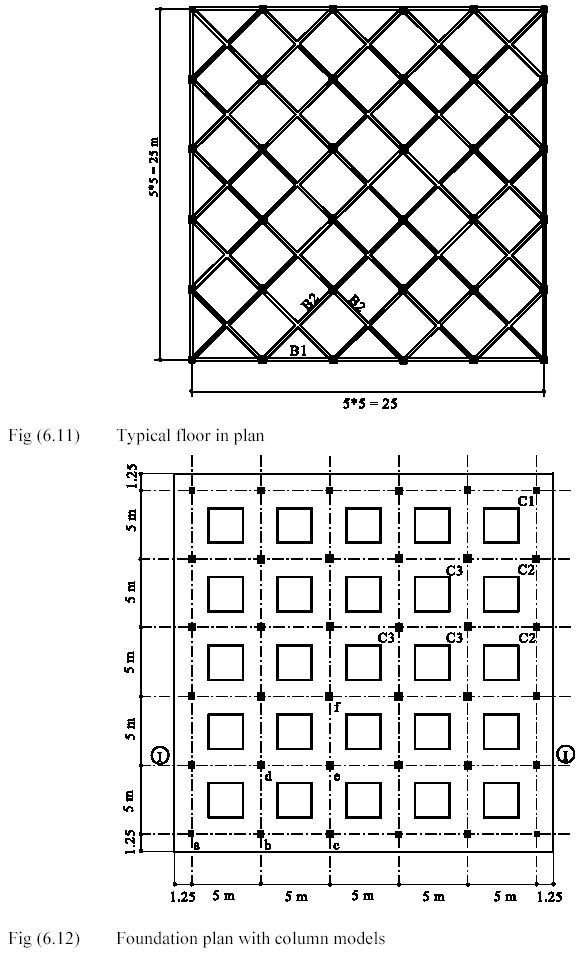Introduction

The presence of the structure on compressible subsoil causes settlements for the foundation and also for the structure itself. Values of settlements and settlement differences depend not only on the thickness of the compressible soil layer under the foundation, the value and distribution of structure loads, the foundation depth and contact pressure under the foundations but also on the flexural rigidity of the structure.

One of the properties that has a considerable influence on the development of settlement is the rigidity of the superstructure. The more rigid structure has more uniform settlement and conversely, structure that is more flexible has greatest difference in settlement. The entire structure can be defined as the three media: superstructure, foundation and soil. The analysis of the entire structure as one unit is very important to find the deformations and internal forces.

However, most of the practical analyses of structures neglect the interaction among the three media to avoid the three-dimensional analysis and modeling. The structure is designed on the assumption of non-displaceable supports while the foundation is designed on the assumption that there is no connection between columns. Such accurate analysis of the entire structure is extremely complex.

The early studies for consideration the effect of the superstructure were by Meyerhof (1953) who suggested an approximate method to evaluate the equivalent stiffness that includes the combined effect of the superstructure and the strip beam foundation. Kany (1959) gave the flexural rigidity of a multi-storey frame structure by an empirical formulae. Also, Kany (1977) analyzed the structure with foundation using a direct method. Demeneghi (1981) used the stiffness method in the structural analysis. Panayotounakos/ Spyropoulos/ Prassianakis (1987) presented an exact matrix solution for the static analysis of a multi-storey and multi-column rectangular plexus frame on an elastic foundation in the most general case of response and loading.

At the analysis of foundations with considering the superstructure stiffness, it is required to distinguish between the analysis for plane structures (two-dimensional analysis) and that for space structures (three-dimensional analysis). Further, it is required to distinguish between approximation methods with closed form equations (Kany (1974), Meyerhof (1953), Sommer (1972)) and refined methods such as conventional plane or space frame analysis (Kany (1976)), Finite Elements (Meyer (1977), Ellner/ Kany (1976), Zilch (1993), Kany/ El Gendy (2000)) or Finite Differences (Bowles (1974), Deninger (1964)).

In addition, many analytical methods are reported for analysis of the entire structure as one unit by using the finite element.

For examples:

Haddadin (1971) presented an explicit program for the analysis of the raft on Winkler's foundation including the effects of superstructure rigidity.

Lee/ Browen (1972) analyzed a plane frame on a two-dimensional foundation.

Hain/ Lee (1974) employed the finite element method to analyze the flexural behavior of a flexible raft foundation taking into account stiffness effect of a framed superstructure. They proposed the use of substructure techniques with finite element formulation to model space frame-raft-soil systems. The supporting soil was represented by either of two types of soil models (Winkler and half-space models).

Poulos (1975) formulated the interaction of superstructure and foundation by two sets of equations. The first set links the behavior of the structure and foundation in terms of the applied structural loads and the unknown foundation reactions. The second set links the behavior of the foundation and underlying soil in terms of the unknown foundation reactions.

Mikhaiel (1978) considered the effect of shear walls and floors rigidity on the foundation.

Bobe/ Hertwig/ Seiffert (1981) considered the plastic behavior of the soil with the effect of the superstructure.

Lopes/ Gusmao (1991) analyzed the symmetrical vertical loading with the effect of the superstructure.

Jessberger/ Yuan/ Thaher/ Ming-bao (1992) considered the effect of the superstructure in case of raft foundation on a group of piles.

Zilch (1993) proposed a method for interaction of superstructure and foundation via iteration.

Kany/ El Gendy (2000) proposed an iterative procedure to consider the effect of superstructure rigidity on the foundation. In the procedure, the stiffness of any substructure such as floor slab or foundation, connected by the columns can be represented by equivalent spring constants due to forces and moments at the connection nodes. Consequently the stiffness matrices of the slab floors, columns and foundation remain unaffected during the iteration process.

Description of problem

To verify the iterative procedure and evaluate its accuracy, a five-storey building resting on foundation through 36 columns is considered. The building is composed of five bays in both x and y-directions, each bay is 5.0 [m] span. The height of the first storey is 4.0 [m] while the height of the other storeys is 3 [m]. The typical floor of the five storeys is chosen to be skew paneled beams as shown in Figure (6.11). The dimensions and loads of floor beams are shown in Table (6.4). The foundation is a grid type with 0.5 [m] thickness and 2.5 [m] breadth, Figure (6.12). The columns are square cross sections, the column models and dimensions for each storey are shown in Table (6.5).

The building material is reinforced concrete and has the following properties:

Young’s modulus Eb = 3×10 [kN/m]

Poisson’s ratio νb = 0.15 

Shear modulus Gb = 1.3×10 [kN/m]

The soil mass below the foundation is idealized as Winkler’s medium. The modulus of subgrade reaction of the soil ks is 40000 [kN/m].

## Example (6.3): Analysis of structure on nonlinear soil medium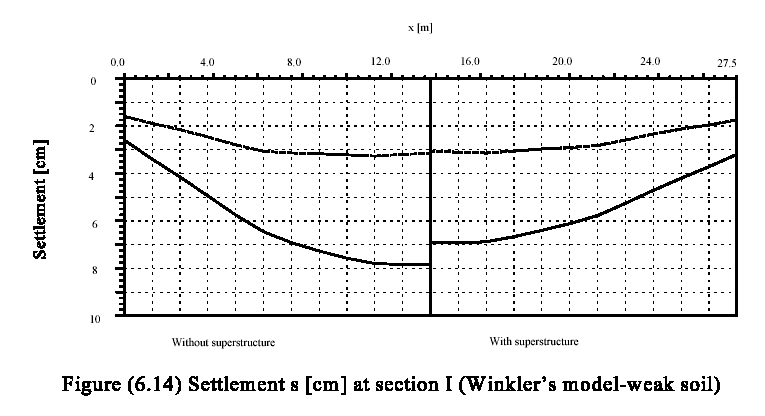Introduction

The presence of the structure on compressible subsoil causes settlements for the foundation and also for the structure itself. Values of settlements and settlement differences depend not only on the thickness of the compressible soil layer under the foundation, the value and distribution of structure loads, the foundation depth and contact pressure under the foundations but also on the flexural rigidity of the structure.

One of the properties that has a considerable influence on the development of settlement is the rigidity of the superstructure. The more rigid structure has more uniform settlement and conversely, structure that is more flexible has greatest difference in settlement. The entire structure can be defined as the three media: superstructure, foundation and soil. The analysis of the entire structure as one unit is very important to find the deformations and internal forces.

However, most of the practical analyses of structures neglect the interaction among the three media to avoid the three-dimensional analysis and modeling. The structure is designed on the assumption of non-displaceable supports while the foundation is designed on the assumption that there is no connection between columns. Such accurate analysis of the entire structure is extremely complex.

The early studies for consideration the effect of the superstructure were by Meyerhof (1953) who suggested an approximate method to evaluate the equivalent stiffness that includes the combined effect of the superstructure and the strip beam foundation. Kany (1959) gave the flexural rigidity of a multi-storey frame structure by an empirical formulae. Also, Kany (1977) analyzed the structure with foundation using a direct method. Demeneghi (1981) used the stiffness method in the structural analysis. Panayotounakos/ Spyropoulos/ Prassianakis (1987) presented an exact matrix solution for the static analysis of a multi-storey and multi-column rectangular plexus frame on an elastic foundation in the most general case of response and loading.

At the analysis of foundations with considering the superstructure stiffness, it is required to distinguish between the analysis for plane structures (two-dimensional analysis) and that for space structures (three-dimensional analysis). Further, it is required to distinguish between approximation methods with closed form equations (Kany (1974), Meyerhof (1953), Sommer (1972)) and refined methods such as conventional plane or space frame analysis (Kany (1976)), Finite Elements (Meyer (1977), Ellner/ Kany (1976), Zilch (1993), Kany/ El Gendy (2000)) or Finite Differences (Bowles (1974), Deninger (1964)).

In addition, many analytical methods are reported for analysis of the entire structure as one unit by using the finite element.

For examples:

Haddadin (1971) presented an explicit program for the analysis of the raft on Winkler's foundation including the effects of superstructure rigidity.

Lee/ Browen (1972) analyzed a plane frame on a two-dimensional foundation.

Hain/ Lee (1974) employed the finite element method to analyze the flexural behavior of a flexible raft foundation taking into account stiffness effect of a framed superstructure. They proposed the use of substructure techniques with finite element formulation to model space frame-raft-soil systems. The supporting soil was represented by either of two types of soil models (Winkler and half-space models).

Poulos (1975) formulated the interaction of superstructure and foundation by two sets of equations. The first set links the behavior of the structure and foundation in terms of the applied structural loads and the unknown foundation reactions. The second set links the behavior of the foundation and underlying soil in terms of the unknown foundation reactions.

Mikhaiel (1978) considered the effect of shear walls and floors rigidity on the foundation.

Bobe/ Hertwig/ Seiffert (1981) considered the plastic behavior of the soil with the effect of the superstructure.

Lopes/ Gusmao (1991) analyzed the symmetrical vertical loading with the effect of the superstructure.

Jessberger/ Yuan/ Thaher/ Ming-bao (1992) considered the effect of the superstructure in case of raft foundation on a group of piles.

Zilch (1993) proposed a method for interaction of superstructure and foundation via iteration.

Kany/ El Gendy (2000) proposed an iterative procedure to consider the effect of superstructure rigidity on the foundation. In the procedure, the stiffness of any substructure such as floor slab or foundation, connected by the columns can be represented by equivalent spring constants due to forces and moments at the connection nodes. Consequently the stiffness matrices of the slab floors, columns and foundation remain unaffected during the iteration process.

Description of problem

An application of the proposed iterative procedure is carried out to study the behavior of foundation resting on nonlinear soil medium with considering influence of the superstructure rigidity.

The previous example shown in Figures. (6.11) and (6.12) is also chosen here to show the analysis of structure on nonlinear soil medium with some modification to be a practical problem.

The floor is chosen to be a slab of 22 [cm] thickness resting on skew paneled beams. The slab carries a uniform load of 11.8 [kN/m2]. Foundation is considered as a raft foundation with openings. The dimensions of paneled beams, columns and foundation are the same as those of the previous example.

Soil properties

Two different types of soil models are considered in this case-study:

i) Winkler’s model that represents the subsoil by isolated springs.

ii) Layered model that considers the subsoil continuum medium.

The foundation is resting on a soil layer of 10 [m], overlying a rigid base. The soil types are represented by the modulus of elasticity, Es, for layered model that yields modulus of subgrade reaction, ks, for Winkler’s model. Table (6.7) shows two different soil types examined in this study according to the soil properties Es and ks. The two soil types are selected to represent weak and stiff soil. Poisson’s ratio is taken νs = 0.3 for the two soil types.

## Example (7.1): Verification of nonlinear analysis for Winkler's model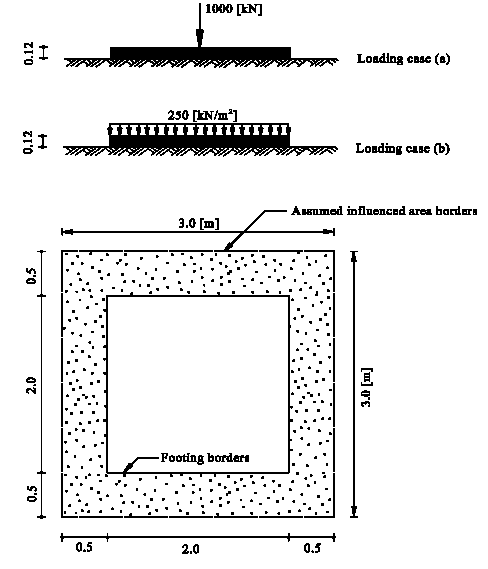Introduction

The simplest model for determination of the contact pressure under the foundation assumes a planar distribution of contact pressure on the bottom of the foundation (statically determined). In which the resultant of soil reactions coincides with the resultant of applied loads. If all contact pressures are compression, the foundation system will be considered as linear and the contact pressures in this case is given directly.

If the foundation subjects to big eccentricity, there will be negative contact pressures on some nodes on the foundation. Since the soil cannot resist negative stress, the foundation system becomes nonlinear and a resolution must be carried out to find the nonlinear contact pressures.

The nonlinear analysis of foundation for the simple assumption model has been treated by many authors since a long time, where several analytical and graphical methods were available for the solution of this problem. Pohl (1918) presented a table to determine the maximum corner pressure max qo for arbitrary positions of the resultant N. Hülsdünker (1964) developed a diagram using the numerical values of this table from Pohl (1918) to determine the maximum corner pressure max qo. For one corner detached footing, the closed form formulae cannot be used. Therefore, Pohl (1918) and Mohr (1918) proposed a method to estimate the neutral axis through the trial and error. Besides tables and diagrams, Graßhoff (1978) introduced also influence line charts can be used to determine the contact pressure ordinates. Peck/ Hanson/ Thornburn (1974) indicated a trial and error method to obtain the neutral axis position for rectangular footing subjected to moments about both axes. Jarquio/ Jarquio (1983) proposed a direct method of proportioning a rectangular footing area subjected to biaxial bending. Irles/ Irles (1994) presented an analytical solution for rectangular footings with biaxial bending, which will lead to obtain explicit solutions for the corner pressures and neutral axis location.

The determination of the actual contact area and the maximum corner pressure max qo under eccentric loaded foundation with irregular shape is very important. For T-shape foundation that is loaded eccentrically in the symmetry axis, Kirschbaum (1970) derived formulae to determine the maximum corner pressure max qo. For some foundation areas with polygonal boundaries, Dimitrov (1977) gave formulae to determine the foundation kern and corner pressure max qo. For the same purpose, Miklos (1964) developed diagrams. For general cases of foundation, Opladen (1958) presented graphical procedure.

Most of the analytical methods used to determine the contact area and corner pressures for eccentric loaded foundations are focused on regular foundations where irregular foundations can be analyzed only by graphical procedures. In this paper, an iteration procedure is presented to deal with nonlinear analysis of foundations for simple assumption model. The procedure can be applied for any arbitrary foundation shape and is suitable for computer programs. The following section describes this procedure.

Description of the problem

To verify the nonlinear analysis of the program ELPLA for Winkler’s soil model, the results of a square footing resting on elastic springs obtained through nonlinear analysis by Hasnien (1993) are compared with those obtained by the program ELPLA. A flexible square footing of 0.12 [m] thickness has the dimensions of 2 [m] × 2 [m] was considered as shown in the Figure.

## Example (7.2): Rectangular foundation subjected to eccentric loading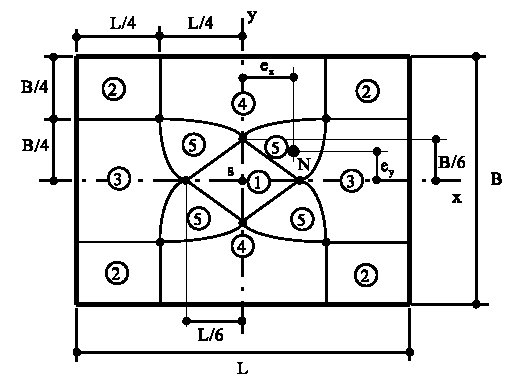Introduction

The simplest model for determination of the contact pressure under the foundation assumes a planar distribution of contact pressure on the bottom of the foundation (statically determined). In which the resultant of soil reactions coincides with the resultant of applied loads. If all contact pressures are compression, the foundation system will be considered as linear and the contact pressures in this case is given directly.

If the foundation subjects to big eccentricity, there will be negative contact pressures on some nodes on the foundation. Since the soil cannot resist negative stress, the foundation system becomes nonlinear and a resolution must be carried out to find the nonlinear contact pressures.

The nonlinear analysis of foundation for the simple assumption model has been treated by many authors since a long time, where several analytical and graphical methods were available for the solution of this problem. Pohl (1918) presented a table to determine the maximum corner pressure max qo for arbitrary positions of the resultant N. Hülsdünker (1964) developed a diagram using the numerical values of this table from Pohl (1918) to determine the maximum corner pressure max qo. For one corner detached footing, the closed form formulae cannot be used. Therefore, Pohl (1918) and Mohr (1918) proposed a method to estimate the neutral axis through the trial and error. Besides tables and diagrams, Graßhoff (1978) introduced also influence line charts can be used to determine the contact pressure ordinates. Peck/ Hanson/ Thornburn (1974) indicated a trial and error method to obtain the neutral axis position for rectangular footing subjected to moments about both axes. Jarquio/ Jarquio (1983) proposed a direct method of proportioning a rectangular footing area subjected to biaxial bending. Irles/ Irles (1994) presented an analytical solution for rectangular footings with biaxial bending, which will lead to obtain explicit solutions for the corner pressures and neutral axis location.

The determination of the actual contact area and the maximum corner pressure max qo under eccentric loaded foundation with irregular shape is very important. For T-shape foundation that is loaded eccentrically in the symmetry axis, Kirschbaum (1970) derived formulae to determine the maximum corner pressure max qo. For some foundation areas with polygonal boundaries, Dimitrov (1977) gave formulae to determine the foundation kern and corner pressure max qo. For the same purpose, Miklos (1964) developed diagrams. For general cases of foundation, Opladen (1958) presented graphical procedure.

Most of the analytical methods used to determine the contact area and corner pressures for eccentric loaded foundations are focused on regular foundations where irregular foundations can be analyzed only by graphical procedures. In this paper, an iteration procedure is presented to deal with nonlinear analysis of foundations for simple assumption model. The procedure can be applied for any arbitrary foundation shape and is suitable for computer programs. The following section describes this procedure.

Description of the problem

For comparison with complex foundation shape, no analytical solution is yet available. Therefore, for judgment on the nonlinear analysis of foundations for simple assumption model, consider the rectangular foundation shown in Figure (7.5). The foundation has the length L = 8.0 [m] and the width B = 6.0 [m]. The foundation carries an eccentric load of N = 2000 [kN].

Both of the x-axis and y-axis are main axes, which intersect in the center of gravity of the foundation area s. The position of resultant N is defined by the ordinates x = ex and y = ey.

Within the rectangle foundation area five zones are represented. It is found that, the contact area and maximum corner pressure max qo depending on the position of the resultant N in these five zones (Irles/ Irles (1994)). In this example, the maximum corner pressure max qo is obtained using the program ELPLA for each zone and compared with other analytical salutations, which are available for rectangular foundation.

## Example (7.3): Circular foundation subjected to eccentric loading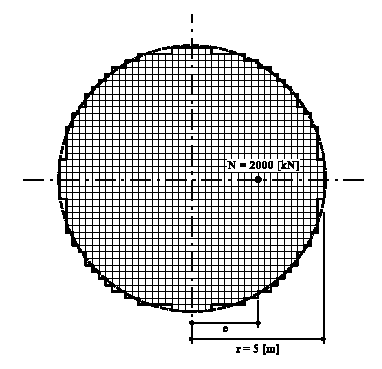Introduction

The simplest model for determination of the contact pressure under the foundation assumes a planar distribution of contact pressure on the bottom of the foundation (statically determined). In which the resultant of soil reactions coincides with the resultant of applied loads. If all contact pressures are compression, the foundation system will be considered as linear and the contact pressures in this case is given directly.

If the foundation subjects to big eccentricity, there will be negative contact pressures on some nodes on the foundation. Since the soil cannot resist negative stress, the foundation system becomes nonlinear and a resolution must be carried out to find the nonlinear contact pressures.

The nonlinear analysis of foundation for the simple assumption model has been treated by many authors since a long time, where several analytical and graphical methods were available for the solution of this problem. Pohl (1918) presented a table to determine the maximum corner pressure max qo for arbitrary positions of the resultant N. Hülsdünker (1964) developed a diagram using the numerical values of this table from Pohl (1918) to determine the maximum corner pressure max qo. For one corner detached footing, the closed form formulae cannot be used. Therefore, Pohl (1918) and Mohr (1918) proposed a method to estimate the neutral axis through the trial and error. Besides tables and diagrams, Graßhoff (1978) introduced also influence line charts can be used to determine the contact pressure ordinates. Peck/ Hanson/ Thornburn (1974) indicated a trial and error method to obtain the neutral axis position for rectangular footing subjected to moments about both axes. Jarquio/ Jarquio (1983) proposed a direct method of proportioning a rectangular footing area subjected to biaxial bending. Irles/ Irles (1994) presented an analytical solution for rectangular footings with biaxial bending, which will lead to obtain explicit solutions for the corner pressures and neutral axis location.

The determination of the actual contact area and the maximum corner pressure max qo under eccentric loaded foundation with irregular shape is very important. For T-shape foundation that is loaded eccentrically in the symmetry axis, Kirschbaum (1970) derived formulae to determine the maximum corner pressure max qo. For some foundation areas with polygonal boundaries, Dimitrov (1977) gave formulae to determine the foundation kern and corner pressure max qo. For the same purpose, Miklos (1964) developed diagrams. For general cases of foundation, Opladen (1958) presented graphical procedure.

Most of the analytical methods used to determine the contact area and corner pressures for eccentric loaded foundations are focused on regular foundations where irregular foundations can be analyzed only by graphical procedures. In this paper, an iteration procedure is presented to deal with nonlinear analysis of foundations for simple assumption model. The procedure can be applied for any arbitrary foundation shape and is suitable for computer programs. The following section describes this procedure.

Description of the problem

Another example is considered to show the applicability of nonlinear analysis of foundations using the program ELPLA for simple assumption model to different foundation types. The results of nonlinear analysis for a circular raft calculated by Teng (1962) are compared with those obtained by the program ELPLA.

A circular raft of radius r = 5 [m] is considered as shown in the Figure. The raft carries an eccentric load of N = 2000 [kN]. The position of the resultant N is defined by the ordinate e.

### About GEOTEC Software

GEOTEC Software is providing universities and consulting companies with the right tools for the last 25+ years. We also assist our clients in various geostructural projects. From basic to advanced problems, GEOTEC Software can provide you with the right technical assistant.